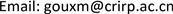1中国电波传播研究所，山东 青岛

2西安电子科技大学，陕西 西安1. 引言

2. 相关干涉仪数学原理

E 1 = A 2 + B 2 + 2 A B cos γ (1)

E 2 = A 2 + B 2 − 2 A B sin γ (2)

.

E 3 = A 2 + B 2 − 2 A B cos γ (3)

E 4 = A 2 + B 2 + 2 A B sin γ (4)

cos γ = E 1 − E 3 4 A B (5)

sin γ = E 4 − E 2 4 A B (6)

e j γ = cos γ + j sin γ = cos γ + j sin γ cos 2 γ + sin 2 γ = ( E 1 − E 3 ) + j ( E 4 − E 2 ) ( E 1 − E 3 ) 2 + ( E 4 − E 2 ) 2 (7)

v = [ e j γ 2 , e j γ 3 , ⋯ , e j γ N ] T (8)

3. 本文提出的单通道相关干涉仪数学原理

E 1 = A 2 + B 2 + 2 A B cos γ (9)

E 5 = A 2 + B 2 + 2 A B cos ( γ + 2 π / 3 ) (10)

E 6 = A 2 + B 2 + 2 A B cos ( γ + 4 π / 3 ) (11)

cos γ = 2 E 1 − E 5 − E 6 6 A B (12)

sin γ = E 6 − E 5 2 3 A B (13)

(14)

Procedure for complex-valued phase vector calculation within the original one-channel correlative interferomete

4. 计算机仿真

Δ = ∑ i 1 = 1 360 ∑ i 2 = 1 8 ∑ i 3 = 1 3000 [ θ ^ 1 ( i 1 , i 2 , i 3 ) − θ ^ 2 ( i 1 , i 2 , i 3 ) ] 2 360 × 8 × 3000 (15)

Procedure for complex-valued phase vector calculation within the proposed one-channel correlative interferomete

Average time consumption ratio and consistency tabl

100 MHz0.7501
200 MHz0.7499
300 MHz0.7498
400 MHz0.7502
500 MHz0.7500
600 MHz0.7501

T 1 = ∑ i 1 = 1 360 ∑ i 2 = 1 8 ∑ i 3 = 1 3000   t 1 ( i 1 , i 2 , i 3 ) 360 × 8 × 3000 (16)

T 2 = ∑ i 1 = 1 360 ∑ i 2 = 1 8 ∑ i 3 = 1 3000   t 2 ( i 1 , i 2 , i 3 ) 360 × 8 × 3000 (17)

5. 结论